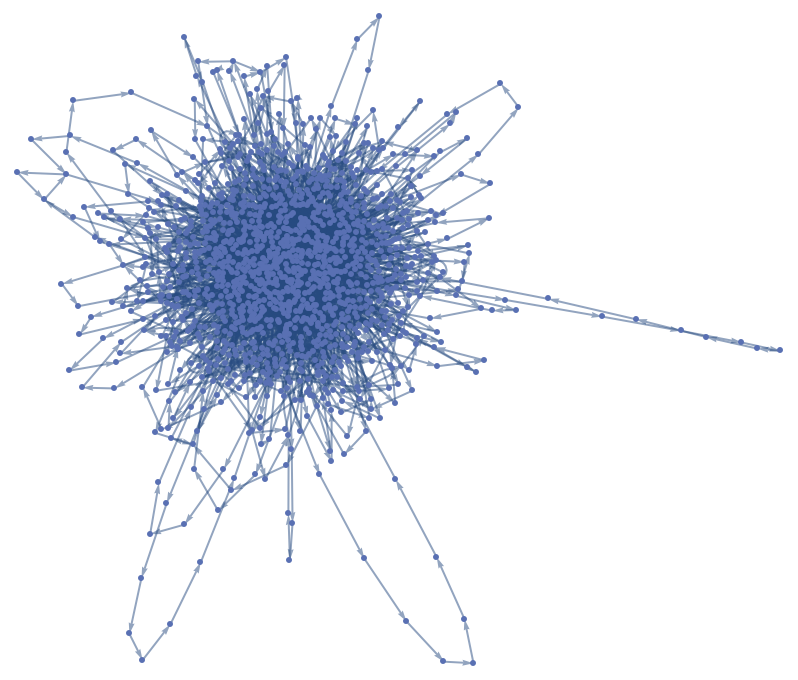#Function Repository Resource:

# SequenceGraph

Create a graph from a data sequence

Contributed by: Daniel de Souza Carvalho
 ResourceFunction["SequenceGraph"][list] creates a graph connecting adjacent entries of list.

## Details and Options

list can contain numbers, strings or symbolic values as input list.
ResourceFunction["SequenceGraph"] accepts the same options as Graph.

## Examples

### Basic Examples

Plot a simple series of numbers:

 In:=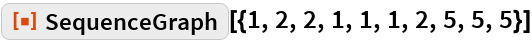Out=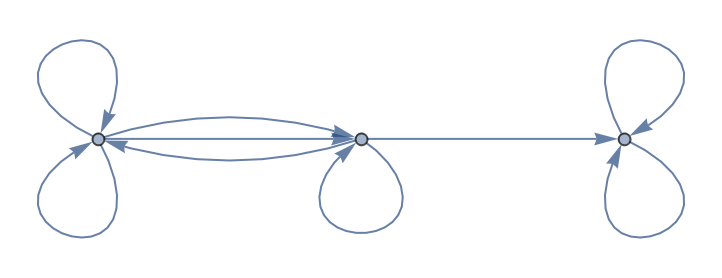SequenceGraph accepts string elements and optional parameters from Graph:

 In:=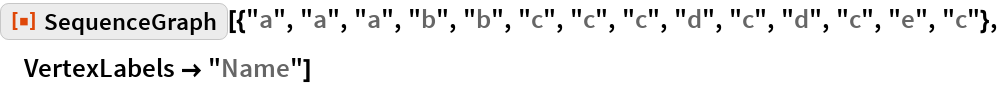Out=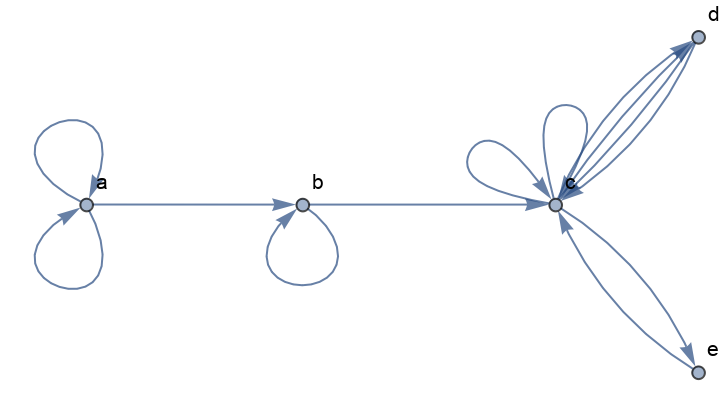SequenceGraph accepts a series of symbolic values:

 In:=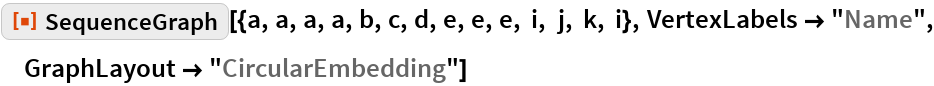Out=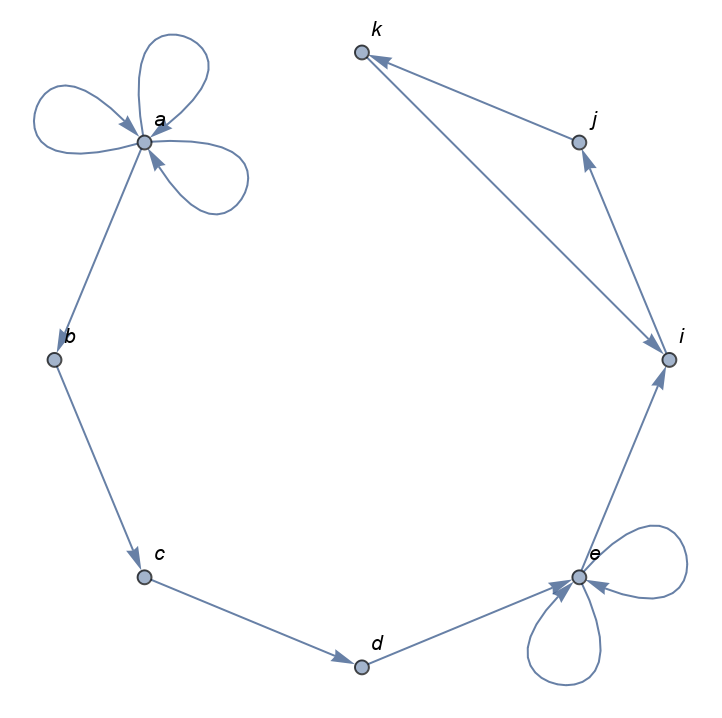Plot a series created by a range of data:

 In:=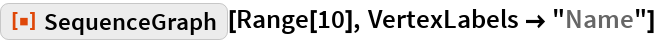Out=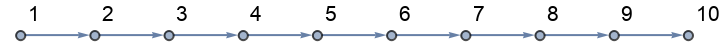Plot the digits of π:

 In:=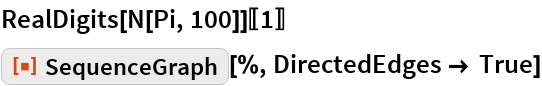Out=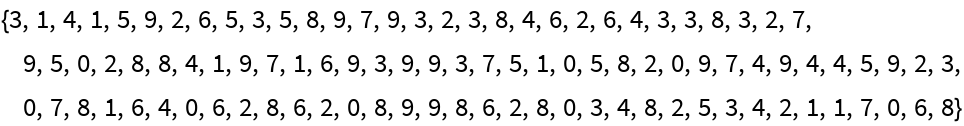Out=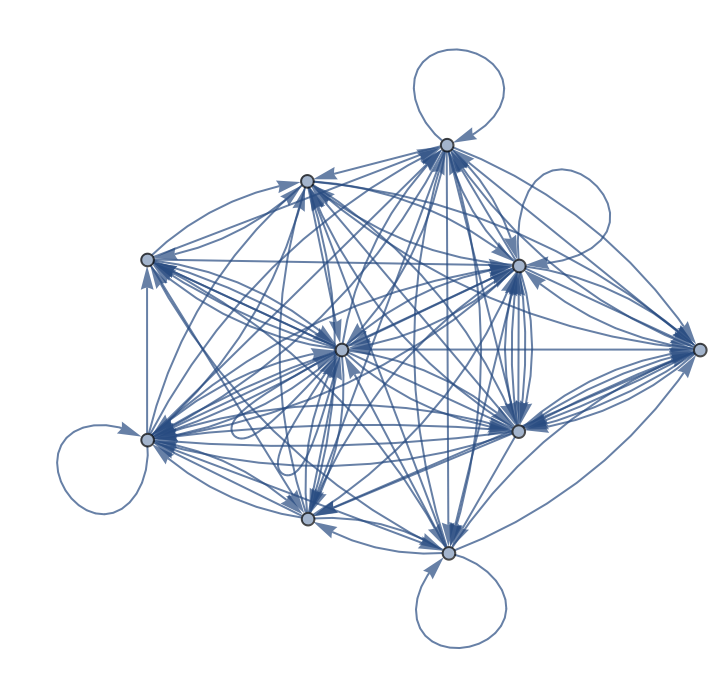Plot the digits of ⅇ:

 In:=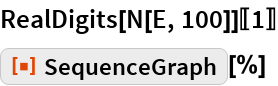Out=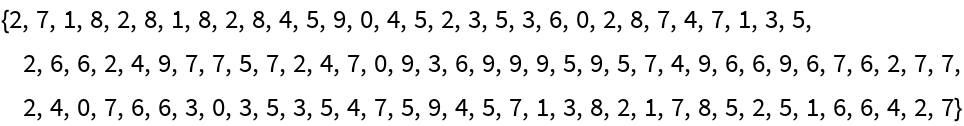Out=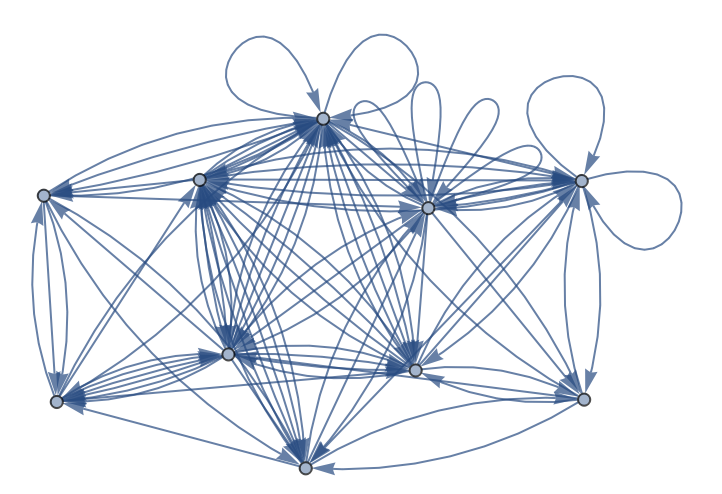### Application

Analyze the dynamical behavior of a class 4 cellular automaton by the sum of black cells of each step, and view as a graph:

 In:=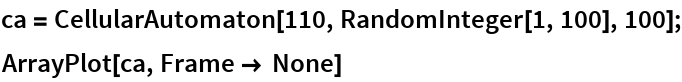Out=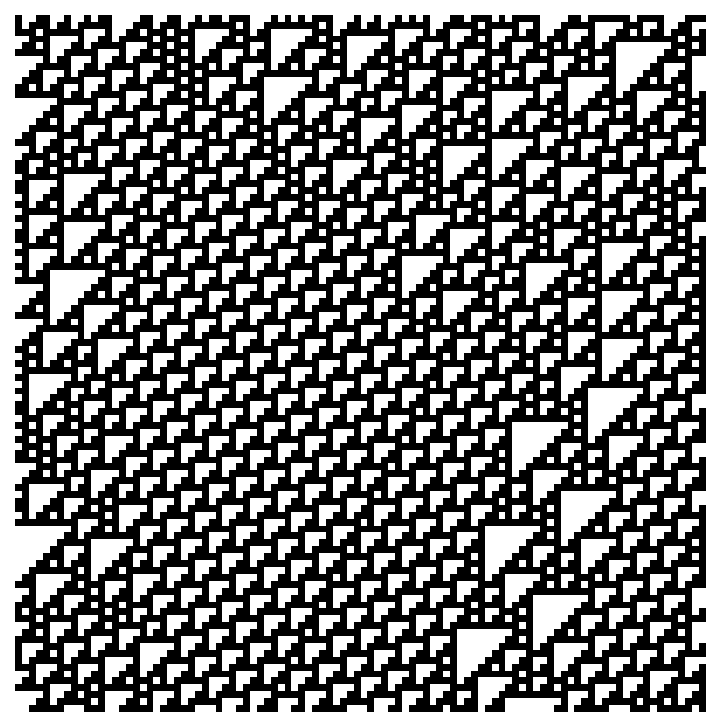In:=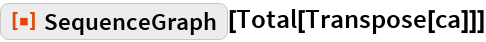Out=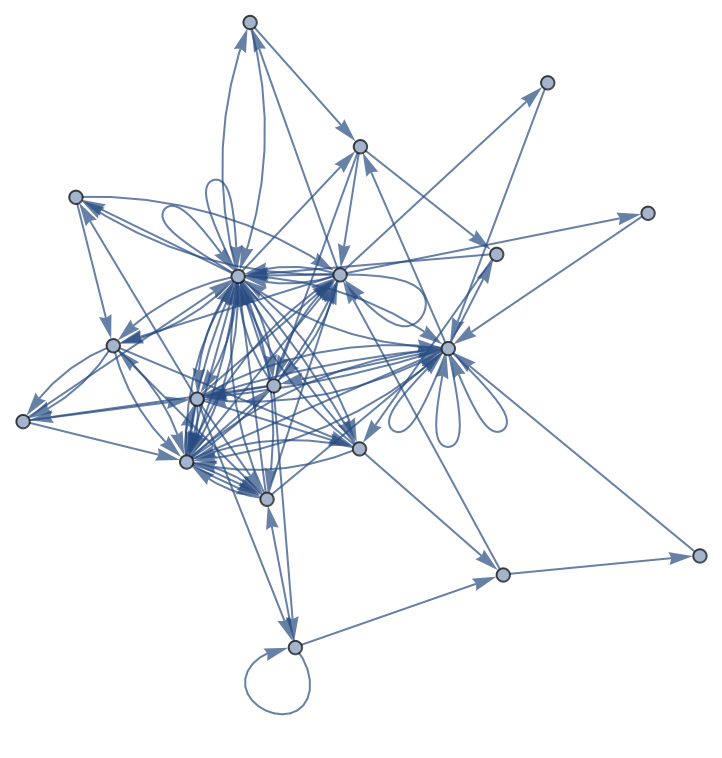Analyze the dynamical behavior of a class 2 cellular automaton by the sum of black cells of each step, and view as a graph:

 In:=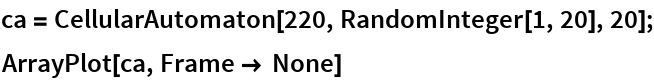Out=In:=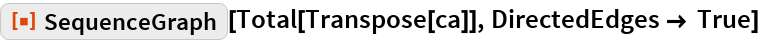Out=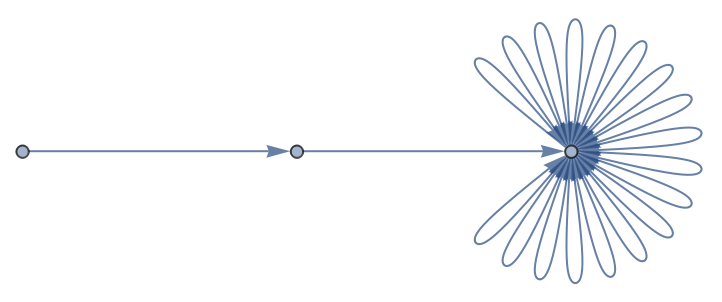Plot the sequence of words in a book:

 In:=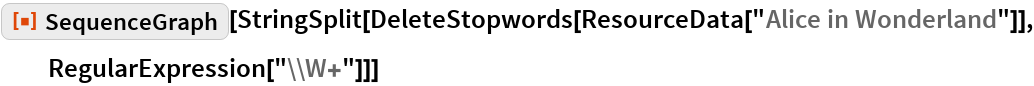Out=